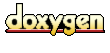Main Page   Namespace List   Compound List   File List   Namespace Members   Compound Members   File Members

TNT::Fortran_Array2D Class Template Reference

`#include <tnt_fortran_array2d.h>`

List of all members.

Public Types

typedef T value_type

Public Methods

Fortran_Array2D ()
Fortran_Array2D (int m, int n)
Fortran_Array2D (int m, int n, T *a)
Fortran_Array2D (int m, int n, const T &a)
Fortran_Array2D (const Fortran_Array2D &A)
Fortran_Array2D& operator= (const T &a)
Fortran_Array2D& operator= (const Fortran_Array2D &A)
Fortran_Array2D& ref (const Fortran_Array2D &A)
Fortran_Array2D copy () const
Fortran_Array2D& inject (const Fortran_Array2D &A)
T& operator() (int i, int j)
const T& operator() (int i, int j) const
int dim1 () const
int dim2 () const
int ref_count () const
~Fortran_Array2D ()

Detailed Description

template<class T> class TNT::Fortran_Array2D

Templated two-dimensional, numerical array which looks like a conventional Fortran array. This is useful when integrating with C/C++ codes translated from Fortran. Storage corresponds to conventional Fortran ordering. That is, the left-most dimension varies fastest. Indexing is via the A(i,j) notation and A(1,1) is the first element.

Array assignment is by reference (i.e. shallow assignment). That is, B=A implies that the A and B point to the same array, so modifications to the elements of A will be reflected in B. If an independent copy is required, then B = A.copy() can be used. Note that this facilitates returning arrays from functions without relying on compiler optimizations to eliminate extensive data copying.

This class employs its own garbage collection via the use of reference counts. That is, whenever an internal array storage no longer has any references to it, it is destoryed.

Member Typedef Documentation

 template typedef T TNT::Fortran_Array2D::value_type

Constructor & Destructor Documentation

 template TNT::Fortran_Array2D< T >::Fortran_Array2D ( )
 Create a null (0x0x0) array.

 template TNT::Fortran_Array2D< T >::Fortran_Array2D ( int m, int n )

Create a new (m x n) array, WIHOUT initializing array elements. To create an initialized array of constants, see Fortran_Array2D(m,n, value).

This version avoids the O(m*n) initialization overhead and is used just before manual assignment.

Parameters:
 m the first dimension of the new matrix. n the second dimension of the new matrix.

 template TNT::Fortran_Array2D< T >::Fortran_Array2D ( int m, int n, T * a )

Create a new (m x n) array, as a view of an existing one-dimensional C array, with elements stored in Fortran order, i.e. right-most dimension varying fastest. (Often referred to as "column-major" ordering.) Note that the storage for this pre-existing array will never be garbage collected by the Fortran_Array2D class.

Parameters:
 m the first dimension of the new matrix. n the second dimension of the new matrix. a the one dimensional C array to use as data storage for the array.

 template TNT::Fortran_Array2D< T >::Fortran_Array2D ( int m, int n, const T & val )

Create a new (m x n ) array, initializing array elements to constant specified by argument. Most often used to create an array of zeros, as in A(m, n, k, 0.0).

Parameters:
 m the first dimension of the new matrix. n the second dimension of the new matrix. val the constant value to set all elements of the new array to.

 template TNT::Fortran_Array2D< T >::Fortran_Array2D ( const Fortran_Array2D & A )` [inline]`
 Copy constructor. Array data is NOT copied, but shared. Thus, in Fortran_Array2D B(A), subsequent changes to A will be reflected in B. For an indepent copy of A, use Fortran_Array2D B(A.copy()), or B = A.copy(), instead.

 template TNT::Fortran_Array2D::~Fortran_Array2D ( )

Member Function Documentation

 template Fortran_Array2D< T > TNT::Fortran_Array2D< T >::copy ( ) const
 Create a new of existing matrix. Used in B = A.copy() or in the construction of B, e.g. Fortran_Array2D B(A.copy()), to create a new array that does not share data.

 template int TNT::Fortran_Array2D< T >::dim1 ( ) const` [inline]`
 Returns: the size of the first dimension of the array.

 template int TNT::Fortran_Array2D< T >::dim2 ( ) const` [inline]`
 Returns: the size of the second dimension of the array.

 template Fortran_Array2D< T > & TNT::Fortran_Array2D< T >::inject ( const Fortran_Array2D & A )

Copy the elements to from one array to another, in place. That is B.inject(A), both A and B must conform (i.e. have identical dimensions).

This differs from B = A.copy() in that references to B before this assignment are also affected. That is, if we have

```	Fortran_Array2D A(m,n);
Fortran_Array2D C(m,n);
Fortran_Array2D B(C);        // elements of B and C are shared.

```
then B.inject(A) affects both and C, while B=A.copy() creates a new array B which shares no data with C or A.
Parameters:
 A the array from elements will be copied
Returns:
an instance of the modifed array. That is, in B.inject(A), it returns B. If A and B are not conformat, no modifications to B are made.

 template const T & TNT::Fortran_Array2D< T >::operator() ( int i, int j ) const` [inline]`
 Read-only version of A(i,j) indexing. If TNT_BOUNDS_CHECK macro is defined, the indices are checked that they falls within the array bounds (via the assert() macro.)

 template T & TNT::Fortran_Array2D< T >::operator() ( int i, int j )` [inline]`
 Elements are accessed via A(i,j) indexing. If TNT_BOUNDS_CHECK macro is defined, the indices are checked that they falls within the array bounds (via the assert() macro.)

 template Fortran_Array2D< T > & TNT::Fortran_Array2D< T >::operator= ( const Fortran_Array2D & A )` [inline]`
 B = A is shorthand notation for B.ref(A).

 template Fortran_Array2D< T > & TNT::Fortran_Array2D< T >::operator= ( const T & a )` [inline]`
 Assign all elemnts of A to a constant scalar.

 template Fortran_Array2D< T > & TNT::Fortran_Array2D< T >::ref ( const Fortran_Array2D & A )` [inline]`
 Create a reference (shallow assignment) to another existing array. In B.ref(A), B and A shared the same data and subsequent changes to the array elements of one will be reflected in the other. This is what operator= calls, and B=A and B.ref(A) are equivalent operations. Returns: The new referenced array: in B.ref(A), it returns B.

 template int TNT::Fortran_Array2D::ref_count ( ) const` [inline]`

The documentation for this class was generated from the following file:
Generated at Thu Jun 26 17:26:24 2003 for Template Numerical Toolkit (TNT) by1.2.5 written by Dimitri van Heesch, © 1997-2001